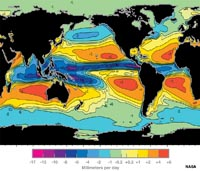# 7.7: Salinity and Latitude

•• Miracosta Oceanography 101
• Miracosta)
$$\newcommand{\vecs}{\overset { \rightharpoonup} {\mathbf{#1}} }$$ $$\newcommand{\vecd}{\overset{-\!-\!\rightharpoonup}{\vphantom{a}\smash {#1}}}$$$$\newcommand{\id}{\mathrm{id}}$$ $$\newcommand{\Span}{\mathrm{span}}$$ $$\newcommand{\kernel}{\mathrm{null}\,}$$ $$\newcommand{\range}{\mathrm{range}\,}$$ $$\newcommand{\RealPart}{\mathrm{Re}}$$ $$\newcommand{\ImaginaryPart}{\mathrm{Im}}$$ $$\newcommand{\Argument}{\mathrm{Arg}}$$ $$\newcommand{\norm}{\| #1 \|}$$ $$\newcommand{\inner}{\langle #1, #2 \rangle}$$ $$\newcommand{\Span}{\mathrm{span}}$$ $$\newcommand{\id}{\mathrm{id}}$$ $$\newcommand{\Span}{\mathrm{span}}$$ $$\newcommand{\kernel}{\mathrm{null}\,}$$ $$\newcommand{\range}{\mathrm{range}\,}$$ $$\newcommand{\RealPart}{\mathrm{Re}}$$ $$\newcommand{\ImaginaryPart}{\mathrm{Im}}$$ $$\newcommand{\Argument}{\mathrm{Arg}}$$ $$\newcommand{\norm}{\| #1 \|}$$ $$\newcommand{\inner}{\langle #1, #2 \rangle}$$ $$\newcommand{\Span}{\mathrm{span}}$$$$\newcommand{\AA}{\unicode[.8,0]{x212B}}$$

## Salinity and Latitude

Figure 7.24 is a map of the globe comparing the rates of evaporation and precipitation. The map is a compilation of evaporation minus precipitation (E-P) values. The data basically shows the regions where there is a net gain of salinity created in surface waters by high evaporation rates. There is also a net loss of salinity where precipitation is higher than evaporation rates (Figure 7.25). In general:

• The tropics (equatorial region) is humid and cloudy, and receives much more rain than evaporates.
• The temperate regions receive less precipitation, so evaporation dominates.
• The polar regions have low evaporation rates relative to the amount of precipitation they receive.Figure 7.24. Map of net evaporation minus precipitation (E-P) on oceans.

 Factors that decrease salinity:Factors that increase salinity:• Precipitation • Runoff • Melting icebergs/sea ice • Evaporation • Freezing sea ice